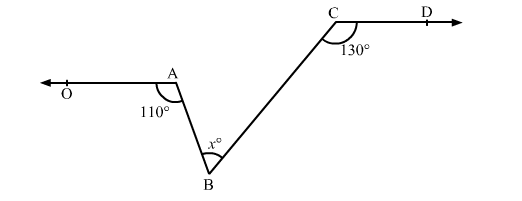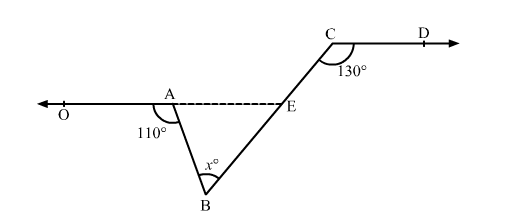# In the given figure, ∠OAB = 110° and ∠BCD = 130° then ∠ABC is equal to

Question:

In the given figure, ∠OAB = 110° and ∠BCD = 130° then ∠ABC is equal to(a) 40°
(b) 50°
(c) 60°
(d) 70°

Solution:

In the given figure, OA || CD.

Construction: Extend OA such that it intersects BC at E.Now, OE || CD and BC is a transversal.

∴ ∠AEC = ∠BCD = 130°       (Pair of corresponding angles)

Also, ∠OAB + ∠BAE = 180°         (Linear pair)

∴ 110° + ∠BAE = 180°

⇒ ∠BAE = 180° − 110° = 70°

In ∆ABE,

∠AEC = ∠BAE + ∠ABE                (In a triangle, exterior angle is equal to the sum of two opposite interior angles)

∴ 130° = 70° + x°

⇒ x° = 130° − 70° = 60°

Thus, the measure of angle ∠ABC is 60°.

Hence, the correct answer is option (c).